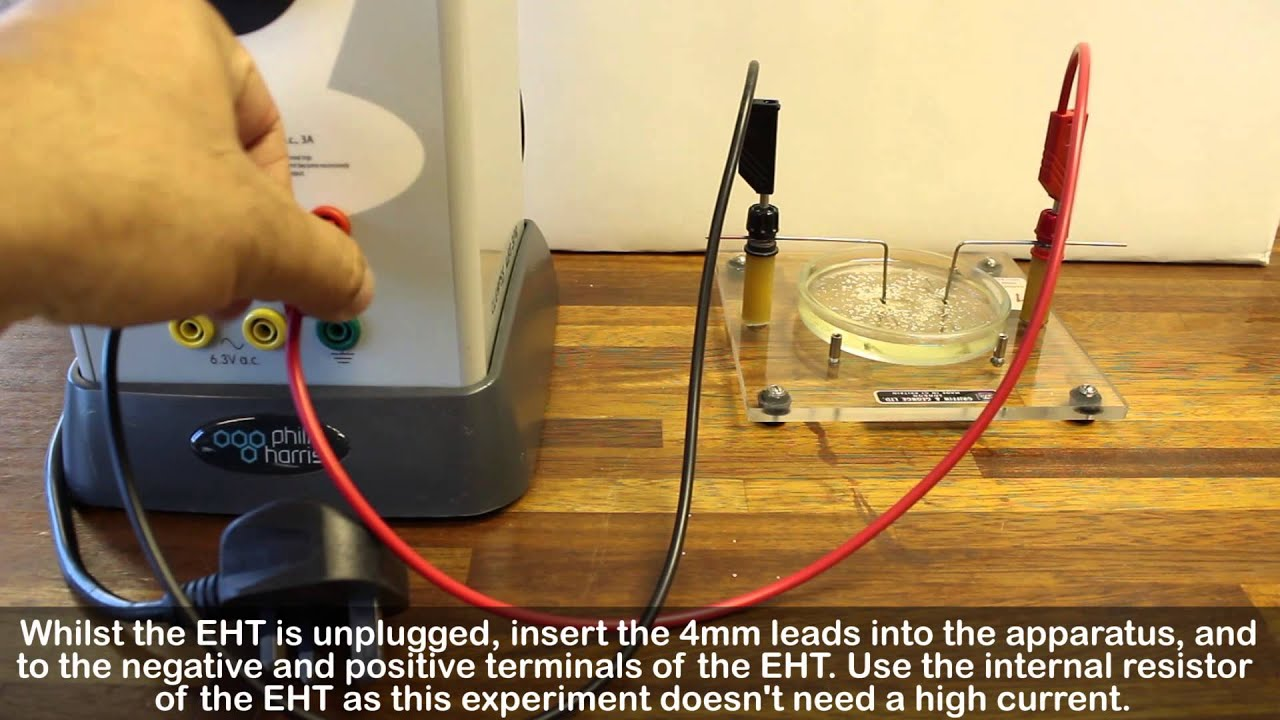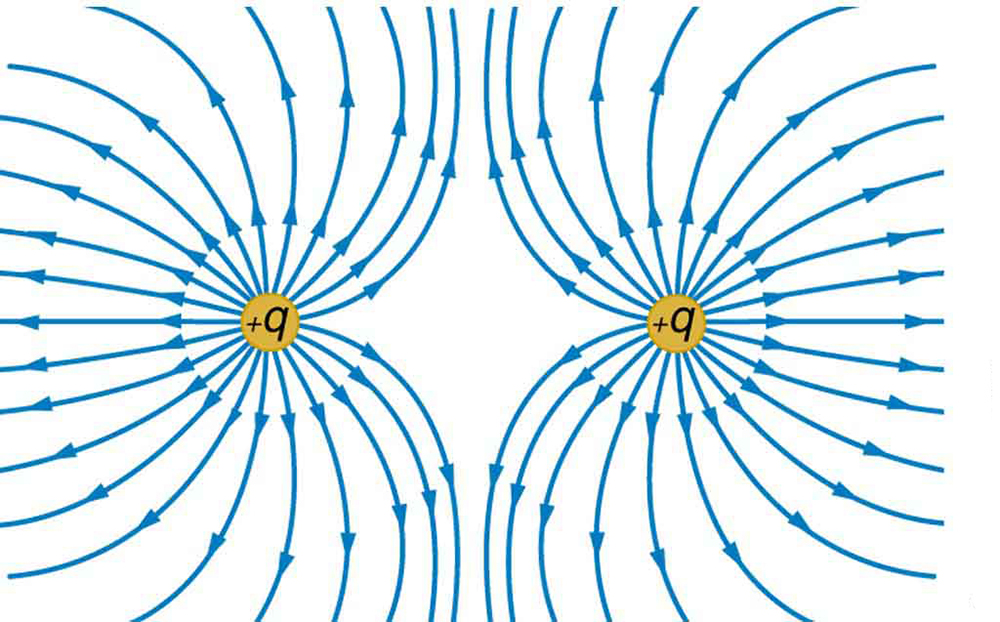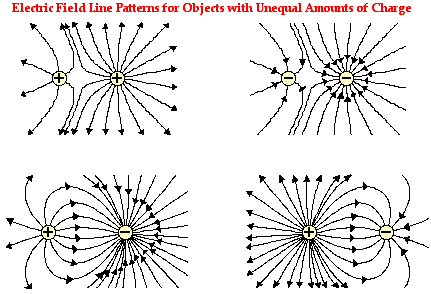Objectives:

• Understand that electric fields are due to charges
• Be able to model a uniformly charged sphere as a point charge at its centre
• Be able to identify and draw electric field lines that can map electric fields

What is a field?

A field in physics is an area that exists when two objects interact with one another via a non-contact force.  Two objects that interact via the gravitational force have a gravitational field that exists between them – the gravitational field is defined as the force per unit mass (any object that has a mass will be influenced by a gravitational field);$g = \frac{F}{m}$, but you are familiar with this as$F = mg$ which comes from$F=ma$ where the acceleration is due to gravity (hence a is changed to a g).

The motion of charged particles are influenced in the same way that two magnets influence one another; two north (like) poles repel whereas a north and a south (opposite) attract. Two of the same charged particles repel one another and if they are of an opposite charge they attract. In other words, two particles that have a negative charge will repel (e.g. two electrons), where two particles with opposite charges will attract (e.g. a proton and an electron).

Since a field is an area that exists when two objects interact with one another via a non-contact force, a field must exist between two charge particles if they are to exert a force on one another (and hence make them repel or attract).

What dies an electric field look like?

Any particle that has an electrical charge will have an electric field associated with it. A positive point charge has field lines that spread out radially from the centre of the point. Negatively charged particles appear the same except the arrows point in the opposite direction.

The field lines are uniform around the charges and always leave the charged particle perpendicular to the surface.

An electric field can be observed using a high voltage power supply connected to two electrodes which are separated by a short distance (in the cm range) and which are placed in some castor oil:An e.m.f. is supplied by the power supply, this then provides a potential difference across the electrodes. This potential difference is due to the charges that are being forced onto the electrodes (one gains some electrons and the others has an absence of electrons).

If semolina seeds are sprinkled around and between the electrodes, they act as mini dipoles (one end of a seed is more positive than the other) and then line up in with the electric field lines.

Watch the following video that shows electric field lines being observed:

You will not need to know how this experiment is carried out but you will need to know what electric field lines look like.

With two opposite charges, the electric field pattern appears as shown:If you look back to figure 1, the field lines come out of a positive point charge and the field lines go in to the negative point charge (both of which can be seen in the image shown here).

The field lines interfere with each other constructively in this case.

With two like charges, the electric field pattern appears as shown:Again, if you look back to figure 1, the field lines come out of a positive point charge and the field lines go in to the negative point charge (both of which can be seen in the image shown here)

The field lines interfere with each other destructively in this case.

What do the electric field lines mean?

• Electric field lines have been defined as the force per unit positive charge,$E = \frac{F}{+Q}$  . This tells us that the electric fields lines represent the direction that an external positively charged particle would be forced to accelerate in if placed at a point in the field.  Since the field lines have arrows the electric field strength,$E$ , must be a vector quantity.
• If a negatively charged particle were to be placed in the field, they would be forced to accelerate in the opposite direction to the fields direction.

If you consider these two bullet points and then scroll back up and look at figures 1, 2 & 3, you should see that if a positively charged particle were placed in the field, it will always be repelled from the fixed positive charged and would be attracted to the negatively charged particles. Similarly, if an electron were placed in the field it would be forced in the opposite direction to the field and hence will be attracted to the fixed positive charge and repelled form the fixed negative charge.

What would the electric field look like between two opposite but different in magnitude charges?

All of the examples above have made the assumption that the two fixed charges have the same charge but opposite sign.  But what if one had a much larger charge than the other?

The larger the charge of a particle, the large the electric field strength and so:Both sets of fields lines need to point inwards (as the charges are negative), the charge on the right has a larger magnitude as there are more field lines.

So simply, we represent a stronger electric field by drawing more field lines on the diagram.

So, going back to original question: But what if one had a much larger charge than the other? The answer is to draw more field lines coming out of the stronger charge than the weaker charge:In this example, charge$q_{1}$  is the stronger of the two charges.

We can also state the$q_{1}$  is negative and$q_{2}$  is positive.

Here are some more examples:http://www.physicsclassroom.com/class/estatics/Lesson-4/Electric-Field-Lines